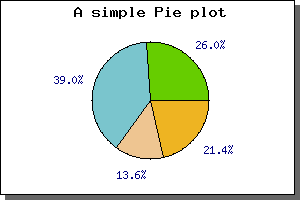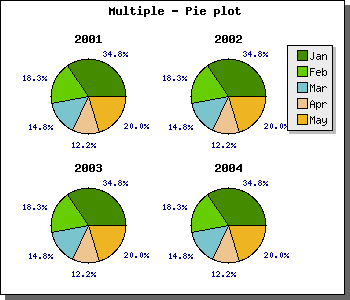Contents Previous Next

## 9.2 Pie plots

So far we have just show plots based on an X-Y coordinate system. This is not the only types of graphs you can create with JpGraph. Another common type is Pie plots. JpGraph supports both 2D and 3D pie plots. For 2D pie plots there are also 2 versions, but more on that later.

The main difference as compared to the X-Y plots is that to all pie plots are added to the PieGraph() instead of the Graph() object we used for the X-Y graphs we have drawn so far. For this you must first include the "jpgraph_pie.php" in your script (or "jpgraph_pie3d.php" if you want to use 3-dimensional pies).

Below you cane see the code needed to create the simplest possible pie graph just using the default settings.

```  include ( "../jpgraph.php"); include ("../jpgraph_pie.php"); \$data  = array(40,60, 21,33); \$graph  = new PieGraph (300,200); \$graph->SetShadow(); \$graph->title-> Set( "A simple Pie plot"); \$p1 = new PiePlot( \$data); \$graph->Add( \$p1); \$graph->Stroke(); ```

The above code would give the following pie graphFigure 117: The simplest possible pie graph [src]

There is a few things worth noting here

• By default all pie slices have the percentage shown just outside the slice.
• The colors are automatically assigned to the slices.
• The pie have the edges marked by default
• The first slice start at 0 degrees (3 o'clock)

You can change almost all aspects of appearance of the pie graphs. For example you could change :

The next simplest addition we can do is to add a legend to the pie graph. We do this by using the SetLegends(); method. By adding the legends to the previous example we get the following imageFigure 118: Adding a legend to the pie graph [src]

(In the figure above we also moved the center of the pie slightly to the left to make more room for the legend box.)

The text for the legends can also contain printf() style format strings to format a number. This number passed on into this string is either the absolute value of the slice or the percentage value. How to switch between the is describe further down in this chapter.

The next change you might want to change is the size and position of the Pie plot. You can change the size with a call to SetSize(); and the position of the center of the pie plot with a call to SetCenter(); The size can be specified as either an absolute size in pixels or as a fraction of width/height (whatever is the smallest). The position of the pie plot is specified as a fraction of the width and height.

To put the size and positioning API to use we will show how to put several pie plots on the same pie graph. In the following example we have also adjusted the legends of the slice values to use a smaller font.

What we do in this example is quite simple, create 4 pie plots, make them smaller and put them in the four corner of the graph. This will give the result as shown in the following example.Figure 119: Multiple pie plots in the same pie graph [src]

Contents Previous Next